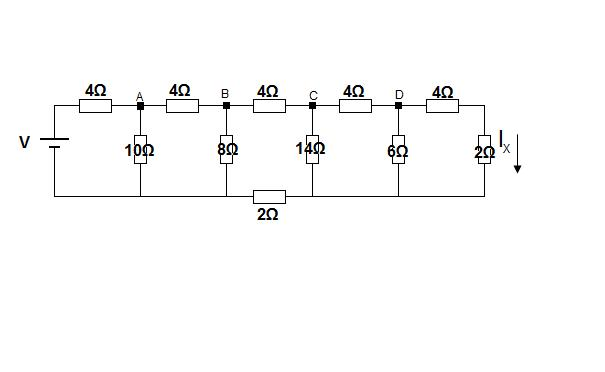#### You may also like### Overarch 2

Bricks are 20cm long and 10cm high. How high could an arch be built without mortar on a flat horizontal surface, to overhang by 1 metre? How big an overhang is it possible to make like this?### Stonehenge

Explain why, when moving heavy objects on rollers, the object moves twice as fast as the rollers. Try a similar experiment yourself.### Maximum Flow

Given the graph of a supply network and the maximum capacity for flow in each section find the maximum flow across the network.

# A Circuit Problem

##### Age 16 to 18 Short Challenge Level:

Starting at the right hand side of the circuit, assume a current of 1A flows in resistor X, and work out the potential drops back to the voltage source. Then use scaling.

Let $I_x$ = 1 amp and find the corresponding V.
We can then use scaling to find $I_x$ when V = 14V.Working right to left:

$I_x$= 1

Find the voltage at d

$V_d = I_x ( 4 + 2)$ = 6V

Find the voltage at c

$V_c = V_d + V_{cd} = V_d + 4I_{cd}$ (Kirchoff's voltage law)

$I_{cd} = I_{d} + I_{x}$ = $\frac{V_d}{6} + 1 = 2$ (Kirchoff's current law)

$V_c = 6V + 8V = 14V$

Find the voltage at b

$V_b= V_c + V_{bc} + (I_x + I_d + I_c)2$

$I_x = 1$
$I_d = \frac{V_d}{6} = 1$
$I_c = \frac{V_c}{14} = 1$

$I_{bc} = I_c + I_{cd} = 3$(Kirchoff's current law)

$V_b = V_c + 4I_{bc} + 6$ = $14 + 12 + 6 = 32V$(Kirchoff's voltage law)

Find the voltage at a

$V_a = V_b + V_{ab} = V_b + 4I_{ab}$

$I_{ab} = I_{bc} + I_b = 3 + \frac{32}{8} = 7$

$V_a = 32 + 28 = 6OV$

Find V when $I_x = 1$

V = $V_a + 4I_{0a}$

$I_{0a} = I_{ab} + \frac{V_a}{10} = 13A$

$V = 60 + 52 = 112V$

We know that in reality V = 14V

All loads are completely resistive, the relationship between voltage and current is therefore linear.

The current which would have given a voltage of 14 V is therefore 1 x $\frac{14}{112}$= 0.125 A

$I_x$ = 0.125 A